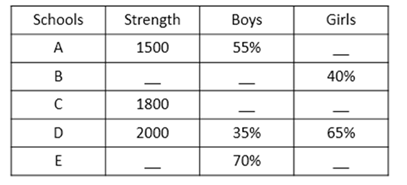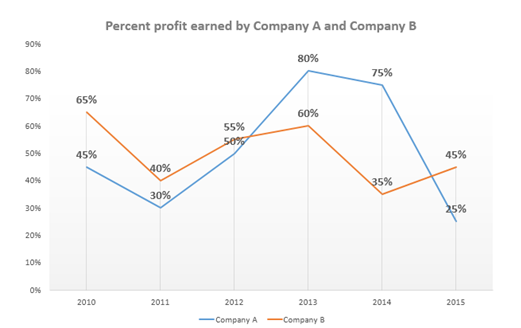# Quantitative Aptitude Questions (Data Interpretation) for SBI Clerk / IDBI Executive 2018 Day-52

Dear Readers, SBI is conducting Online preliminary Examination for the recruitment of Clerical Cadre. preliminary Examination of SBI Clerk was scheduled from June/July 2018. To enrich your preparation here we have providing new series of Data Interpretation – Quantitative Aptitude Questions. Candidates those who are appearing in SBI Clerk Prelims and IDBI Executive Exams can practice these Quantitative Aptitude average questions daily and make your preparation effective.

Click “Start Quiz” to attend these Questions and view Solutions

Directions (Q. 1 – 5): Study the following information carefully and answer the questions given below:

The following table represents strength of five different schools and the percentage of boys and girls in those schools.1. Number of girls in school A is what percent of the number of girls in school D?

a) 61.29 %

b) 51.92 %

c) 55.24 %

d) 65.35 %

e) 48.78 %

1. If the strength of school B is 2500 and the strength of school E is 3500, number of girls of school E is what percent more than the number of girls of school B?

a) 8%

b) 6%

c) 5%

d) 10%

e) None of these.

1. If the ratio of number of boys and number of girls of school C is 5:1 respectively, find the sum number of boys of school C and number of boys of school D.

a) 2500

b) 1500

c) 1000

d) 2200

e) None of these

1. If the strength of school B is 2100, find the respective ratio of number of girls of school B and number of girls of school A.

a) 56:45

b) 45:56

c) 46:45

d) 45:46

e) None of these

1. Strength of schools A and C together is what percent more than the strength of school D?

a) 35%

b) 65%

c) 55%

d) 45%

e) None of these

Directions (Q. 6 – 10): Study the following information carefully and answer the questions given below:

The following line graph represents percent profit earned by two companies over six years.1. If the expenditure of company A in 2010 is Rs.60000, and company B in 2013 is Rs.150000, then find the sum of their incomes.

a) Rs.245000

b) Rs.327000

c) Rs.325000

d) Rs.225000

e) None of these

1. If the incomes of company A and company B are same in 2014, find the ratio of their respective expenditures.

a) 7:5

b) 27:35

c) 35:27

d) 5:7

e) None of these

1. Total percent profit earned by company A over all the years is what percent of total percent profit earned by company B over all the years?

a) 102.67%

b) 93.43%

c) 101.67%

d) 103.76%

e) None of these

1. If the expenditure of company A and company B in 2012 are Rs.75000 and Rs.120000 respectively, Income of company B is what percent more than the income of company A in 2012?

a) 68.53%

b) 53.66%

c) 63.53%

d) 65.33%

e) None of these

1. Which of the following statement is true about company A?

a) Profit earned by company A is maximum in 2013.

b) Profit earned by company A is minimum in 2015.

c) Profit earned by company A is more than that of company B in 2011

d) Can’t be determined

e) None of these.

Directions (Q. 1 – 5):

Percentage of girls in school A = 100 – 55 = 45%

Number of girls in school A = 45/100 x 1500 = 675

Number of girls in school D = 65/100 x 2000 = 1300

Required percentage = 675/1300 x 100 = 51.92%.

Percentage of girls of school E = (100 – 70) = 30%

Number of girls of school E = 30/100 x 3500 = 1050

Number of girls of school B = 40/100 x 2500 = 1000

Required percentage = [(1050 – 1000)/1000] x 100 = 50/1000 x 100 = 5%

Number of boys of school C = 5/6 x 1800 = 1500

Number of boys of school D = 35/100 x 2000 = 700

Required sum = 1500 + 700 = 2200.

Number of girls of school B = 40/100 x 2100 = 840

Number of girls of school A = (100 – 55)/100 x 1500 = 675

Required ratio = 840 : 675 = 56 : 45

Strength of schools A and C together = 1500 + 1800 = 3300

Required percentage = [(3300 – 2000)/2000] x 100 = 65%

Directions (Q. 6 – 10):

Income of company A in 2010 = 145/100 x 60000 = 87000

Income of company B in 2013 = 160/100 x 150000 = 240000

Required sum = 87000 + 240000 = Rs.327000

Let income of company A and B be Rs. x in 2014

Income (A) = exp (A) * 175/100

Exp (A) = income (A) * 100/175 = x * 100/175

Income (B) = exp (B) * 135/100

Exp (B) = income (B) * 100/135 = x * 100/135

Exp (A)/exp (B) = (x * 100/175)/(x * 100/135) = 135/175 = 27:35

Total percent profit earned by company A over all the years

= 45 + 30 + 50 + 80 + 75 + 25 = 305

Total percent profit earned by company B over all the years

= 65 + 40 + 55 + 60 + 35 + 45 = 300

Required percentage = (305/300) x 100 = 101.67%

Income of company A in 2012 = 75000 x 150/100 = 112500

Income of company B in 2012 = 120000 x 155/100 = 186000

Required percentage = (186000 – 112500)/112500 x 100

= [73500/112500] x 100

= 65.33%

We cannot say profit earned by company is more or less in any year because only profit % is given and without knowing the expenditure, this cannot be determined.

Daily Practice Test Schedule | Good Luck

 Topic Daily Publishing Time Daily News Papers & Editorials 8.00 AM Current Affairs Quiz 9.00 AM Logical Reasoning 10.00 AM Quantitative Aptitude “20-20” 11.00 AM Vocabulary (Based on The Hindu) 12.00 PM Static GK Quiz 1.00 PM English Language “20-20” 2.00 PM Banking Awareness Quiz 3.00 PM Reasoning Puzzles & Seating 4.00 PM Daily Current Affairs Updates 5.00 PM Data Interpretation / Application Sums (Topic Wise) 6.00 PM Reasoning Ability “20-20” 7.00 PM English Language (New Pattern Questions) 8.00 PM General / Financial Awareness Quiz 9.00 PM